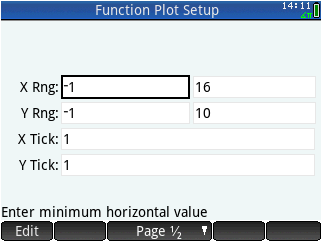# HP Forums

Full Version: plotting time discrete data
You're currently viewing a stripped down version of our content. View the full version with proper formatting.
Hi everybody!

I have a vector of time-discrete data. How do I plot a one dimensional vector?
Is there an app that does the Job or a smart way?
Either simply dots at the sampled Points or a polyline (implied polyline would be great anyway)?

say M1:=[0,1,2,3,4,0,1,2,3,4,0,1,2,3,4]; is a sampled sawtooth where the index into the vector is supposed to be my sample index. No absolute time needed, just implied tn.
You can use the "Statistics 1Var" app, after selecting it you need to move your data to the D1 list:
[attachment=2200]
then in the Symb view select Line as the type of Plot:
[attachment=2201]
and with Plot you can view your data:
[attachment=2202]
I will present some results of my work. Not nearly satisfactional....

I decided to make a polyline(M) function that accepts a 1-dimensional vector as argument. It should use LINE_P() for plotting a polyline. That sounds nice, but I have the Feeling that i am stuck.
For my general understanding: LINE_P accepts a Vector of Points, i.e. vectors with 2 entries for the 2-dimensional Problems and a Vector of indices into the above buffer that define line segments. Similar to a vbo I think?

Help or an example on LINE_P with Point lists would be great!

second problem: when trying to convert my 1-dim vector into a points array I fail.
Code:
``` EXPORT polyline(data) BEGIN LOCAL v_data := MAKEMAT(I,length(data),2); v_data(2):=TRN(data); //How to assign? What should the Points Array look like? //LOCAL l_data:={{1,2},{2,3},{3,4},{4,5},{5,6}}; //How can I build such a vector in Dependance of length(data)? And row/column? Which format //Will deal with that later....... //RECT(); //LINE_P(v_data,l_data); //FREEZE; RETURN v_data; //Output for debugging END;```

How can assign a column in v_data? I realize that I am totally confused with row- and column vectos AND I do not know the best way to manipulate either with my prime.

Thanks a lot for your help. It will lead to a working function, hopefully!
Thanks Didier!

However maybe you can help me getting my polyline() function working? It is a good way to learn.
(06-23-2015 02:11 PM)leprechaun Wrote: [ -> ]Thanks Didier!

However maybe you can help me getting my polyline() function working? It is a good way to learn.

Here is a very rough implementation of the polyline() function, you'll have to adjust the pixel mapping to your needs:

Code:
```EXPORT polyline(data) BEGIN   LOCAL x_max, y_max;   LOCAL L_Points, L_Lines;   x_max:= length(data); y_max:=MAX(data);   L_Points := MAKELIST({IP(I*300/x_max)+10, 220-IP(data(I)*220/y_max)+10},I,1,x_max);   L_Lines := MAKELIST({I,I+1},I,1, x_max-1);   RECT();   LINE_P(10,230,320,230); LINE_P(10,10,10,230);   // Draw X & Y Axis   LINE_P(L_Points,L_Lines,[[1,0],[0,1]]);   FREEZE;   RETURN L_Points; //Output for debugging END;```
Here is another rather simple way using the Function app:Reference URL's
• HP Forums: https://www.hpmuseum.org/forum/index.php
• :